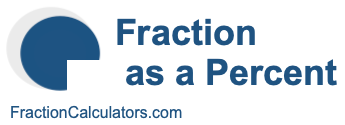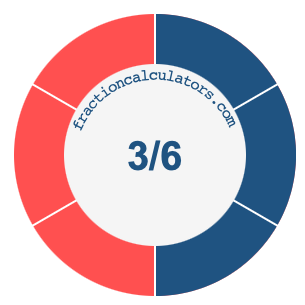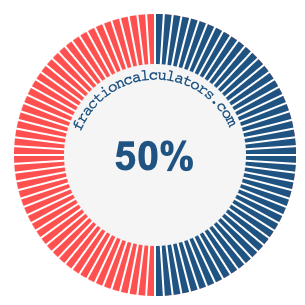3/6 as a percentWhat is 3/6 as a percent? Here we will explain and show you how to calculate and convert 3/6 to a percent.

In addition, we will also illustrate the fraction 3/6 on a pie chart and 3/6 as a percent on a pie chart.

3/6 is a fraction that means 3 out of 6, and percent means something out of 100. We can therefore make the following equation to get 3/6 as a percent:

 3 6
=
 percent 100

To find the percent in the equation above, we multiply 3 by 100 and then divide the product by 6. Here is the solution to the equation above and the answer to 3/6 as a percent:

 3 6
=
 50 100

3/6 = 50%

The illustrations below show you how 3/6 and 50% divide a pie chart differently, but cover the same area because they are the same.Fraction as a Percent Calculator
You can use this tool to convert another fraction to percent.

/

3/7 as a percent
Here is the next fraction on our list that we have converted to a percent for you.